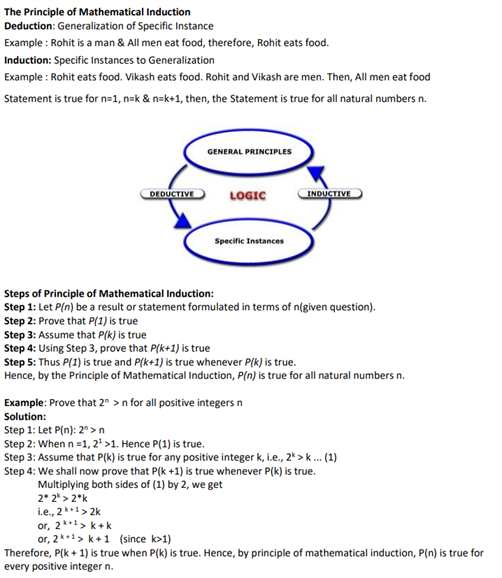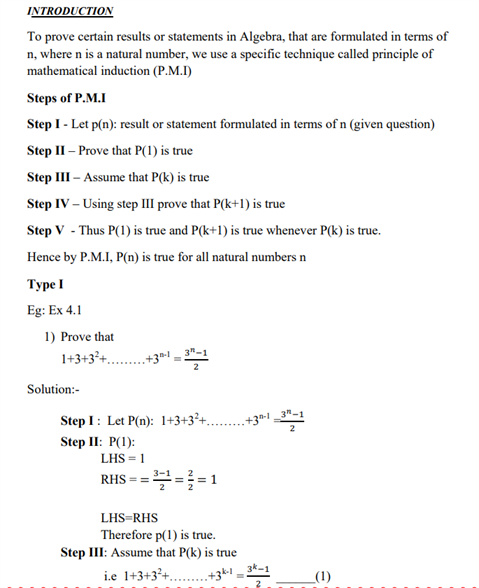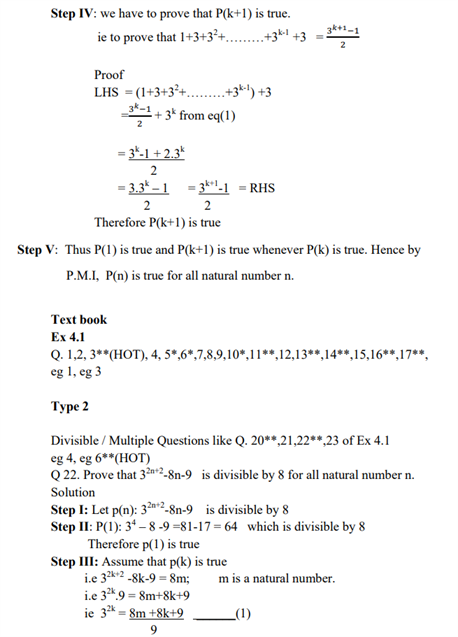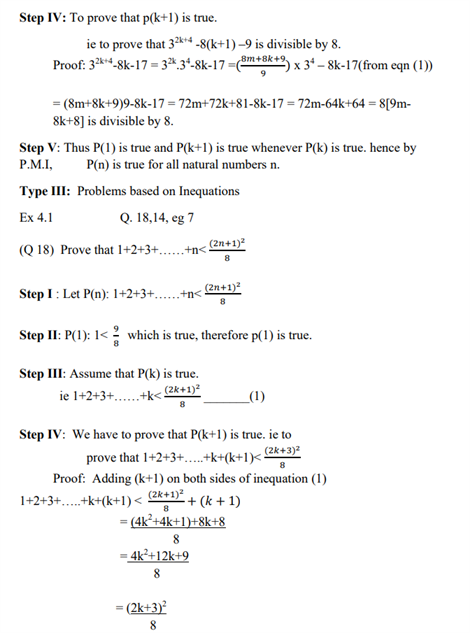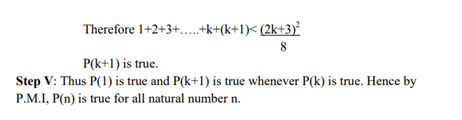# Revision Notes For CBSE Class 11 Maths Chapter 4 Principles Of Mathematical Induction

Class 11 Maths Revision Notes Chapter 4 Principles Of Mathematical Induction consists of all the important theories and explanations related to this very important chapter in maths. Let’s understand what do we mean by mathematical induction:

• Mathematical induction is a specialized form of deductive reasoning used to prove a fact about all the elements in an infinite set by performing a finite number of steps.
• In order for mathematical induction to work with an infinite set, that set must be denumerable, meaning that a one-to-one correspondence must exist between the elements of the set in question and the set of positive integers. In other words, it must be possible to express the set in the form an implied list of discrete elements such as {1, 2, 3, 4, …}.

These notes will provide a helping hand when preparing for exams. Here, students can find the definition of important concepts such as deduction and induction. Viewers will also be witnessing steps of principle of mathematical induction etc. The information in notes is systematically arranged for utmost convenience.

Check out the Class 11 maths revision notes Chapter 4 Principles of Mathematical Induction below